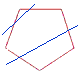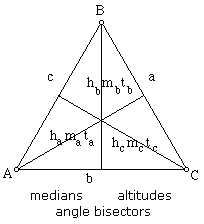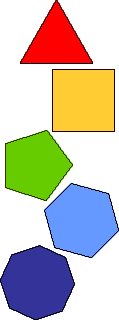Types of Polygons
 Polygon - Closed figure madeup of several linbe   segments that are joined together. The sides do not cross each other. Exactly two sides meet at every vertexRegular Polygon - when all angles and all sides of the polygon are of equal lengthEquilateral Polygon - A polygon when are the sides are the same length.Equiangular Polygon - is a polygon with all congruent angles.Convex polygon - a polygon in which no segment connecting two vertices is outside the polygon.   Concave polygon- a polygon in which at least one segment connecting two vertices is outside the polygon.